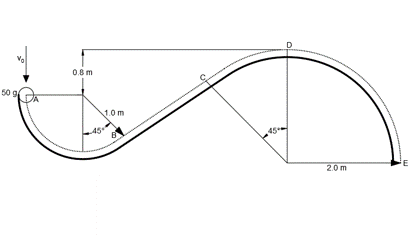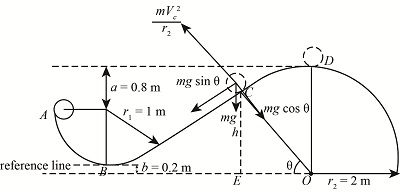# A 50-g ball is released onto a platform at A with initial speed v0. The dotted line ABCDE tracks...

## Question:

A 50-g ball is released onto a platform at A with initial speed v0. The dotted line ABCDE tracks the centre of the ball. Segment AB is a circular are of radius 1.0 m, Segment BC is a straight line and segment CDE is a circular are of radius 2.0 m. Gravity points down.

(a) Determine the minimum initial speed vo so that the ball clears the highest point of the platform at D.

(b) Determine the minimum initial speed to so that the ball leaves the platform at C.## Conservation of energy

As per this law the energy of a system will always remains constant or conserved which means the energy of a system can never be generated or can't be destroyed.

## Answer and Explanation:

(a)

Given Data:

• The mass of the ball is: {eq}m = 50\;{\rm{g}} {/eq}.
• The value of angle is: {eq}\theta = 45^\circ {/eq}.

The figure below shows the free body diagram of the ball.The expression of the kinetic energy of the ball at point A is,

{eq}{K_{\rm{A}}} = \dfrac{1}{2}mV_0^2 {/eq}

The expression for the potential energy of the ball at point A is,

{eq}{P_{\rm{A}}} = mg\left( {{r_1}} \right) {/eq}

As the ball reaches its maximum height at point D, its velocity will be zero.

Therefore, {eq}{V_{\rm{D}}} = 0\;{{\rm{m}} {\left/ {\vphantom {{\rm{m}} {\rm{s}}}} \right. } {\rm{s}}} {/eq}.

The expression of the kinetic energy of the ball at point D is,

{eq}{K_{\rm{D}}} = \dfrac{1}{2}mV_D^2 {/eq}

The expression for the potential energy of the ball at point D is,

{eq}{P_{\rm{D}}} = mg\left( {{r_1} + 0.8\;{\rm{m}}} \right) {/eq}

The expression for the conservation of energy of the ball between A to D is,

{eq}{K_{\rm{A}}} + {P_{\rm{A}}} = {K_{\rm{D}}} + {P_{\rm{D}}} {/eq}

Substitute the values in above expression.

{eq}\begin{align*} \dfrac{1}{2}mV_0^2 + mg\left( {{r_1}} \right) &= \dfrac{1}{2}mV_D^2 + mg\left( {{r_1} + 0.8\;{\rm{m}}} \right)\\ {V_{\rm{0}}} &= \sqrt {2\left( {9.81\;{{\rm{m}} {\left/ {\vphantom {{\rm{m}} {{{\rm{s}}^2}}}} \right. } {{{\rm{s}}^2}}}} \right)\left( {0.8\;{\rm{m}}} \right)} \\ {V_0} &= 3.9618\;{{\rm{m}} {\left/ {\vphantom {{\rm{m}} {\rm{s}}}} \right. } {\rm{s}}} \end{align*} {/eq}

Thus the initial velocity of the ball is {eq}3.9618\;{{\rm{m}} {\left/ {\vphantom {{\rm{m}} {\rm{s}}}} \right. } {\rm{s}}} {/eq}.

(b)

The expression for the equilibrium of force perpendicular to the curve path is,

{eq}\begin{align*} \dfrac{{mV_{\rm{c}}^2}}{{{r_2}}} - mg\cos \theta &= 0\\ {V_{\rm{c}}} &= \sqrt {g{r_2}\cos \theta } \end{align*} {/eq}

Substitute the values in above expression.

{eq}\begin{align*} {V_{\rm{c}}} &= \sqrt {\left( {9.81\;{{\rm{m}} {\left/ {\vphantom {{\rm{m}} {{{\rm{s}}^2}}}} \right. } {{{\rm{s}}^2}}}} \right)\left( {2\;{\rm{m}}} \right)\cos \left( {45^\circ } \right)} \\ &= 3.724\;{{\rm{m}} {\left/ {\vphantom {{\rm{m}} {\rm{s}}}} \right. } {\rm{s}}} \end{align*} {/eq}

The expression of the kinetic energy of the ball at point A is,

{eq}{K_{\rm{A}}} = \dfrac{1}{2}mV_0^2 {/eq}

The expression for the potential energy of the ball at point A is,

{eq}{P_{\rm{A}}} = mg\left( {{r_1}} \right) {/eq}

The expression of the kinetic energy of the ball at point C is,

{eq}{K_{\rm{C}}} = \dfrac{1}{2}mV_c^2 {/eq}

The expression for the potential energy of the ball at point C is,

{eq}{P_{\rm{C}}} = mg\left( {{r_2}\cos \theta - 0.2\;{\rm{m}}} \right) {/eq}

The expression for the conservation of energy of the ball between A to D is,

{eq}{K_{\rm{A}}} + {P_{\rm{A}}} = {K_{\rm{C}}} + {P_{\rm{C}}} {/eq}

Substitute the values in above expression.

{eq}\begin{align*} \dfrac{1}{2}mV_0^2 + mg\left( {{r_1}} \right) &= \dfrac{1}{2}mV_c^2 + mg\left( {{r_2}\cos \theta - 0.2\;{\rm{m}}} \right)\\ {V_{\rm{0}}} &= \sqrt {V_c^2 + 2g\left( {{r_2}\cos \theta - 0.2\;{\rm{m}} - {r_1}} \right)} \\ {V_0} &= \sqrt {{{\left( {3.724\;{{\rm{m}} {\left/ {\vphantom {{\rm{m}} {\rm{s}}}} \right. } {\rm{s}}}} \right)}^2} + 2\left( {9.81\;{{\rm{m}} {\left/ {\vphantom {{\rm{m}} {{{\rm{s}}^2}}}} \right. } {{{\rm{s}}^2}}}} \right)\left( {\left( {2\;{\rm{m}}} \right)\cos \left( {45^\circ } \right) - 0.2\;{\rm{m}} - 1\;{\rm{m}}} \right)} \\ {V_0} &= 4.250\;{{\rm{m}} {\left/ {\vphantom {{\rm{m}} {\rm{s}}}} \right. } {\rm{s}}} \end{align*} {/eq}

Thus the initial velocity of the ball is {eq}4.250\;{{\rm{m}} {\left/ {\vphantom {{\rm{m}} {\rm{s}}}} \right. } {\rm{s}}} {/eq}.

#### Learn more about this topic:Energy Conservation and Energy Efficiency: Examples and Differences

from Geography 101: Human & Cultural Geography

Chapter 13 / Lesson 9
100K